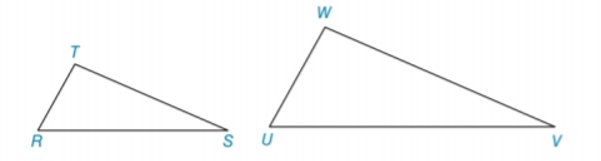Chapter 5.3, Problem 8EElementary Geometry For College St...

7th Edition
Alexander + 2 others
ISBN: 9781337614085

Solutions

Chapter
SectionElementary Geometry For College St...

7th Edition
Alexander + 2 others
ISBN: 9781337614085
Textbook Problem

In Exercises 5 to 8, name the method ( A A , S S S ~ ,   o r   S A S ~ ) that is used to show that the triangles are similar. T R W U = T S W V = R S U VTo determine

To name:

The method that is used to show that the triangles are similar.

Explanation

Given:

The triangles,

TRWU=TSWV=RSUV

Approach:

Two polygons are similar if and only if two conditions are satisfied.

1. All pairs of corresponding angles are congruent.

2. All pairs of corresponding sides are proportional.

Definitions:

AA:

If the two angles of one triangle are congruent to the two angles of a another triangle, then the triangles are similar.

SAS:

If an angle of one triangle is congruent to an angle of a second triangle and the pairs of sides including the angles are proportional (in length), then the triangles are similar

Still sussing out bartleby?

Check out a sample textbook solution.

See a sample solution

The Solution to Your Study Problems

Bartleby provides explanations to thousands of textbook problems written by our experts, many with advanced degrees!

Get Started

In Exercises 31-34, evaluate h(2), where h = g f. 31. f(x) = x2 + x + 1; g(x) = x2

Applied Calculus for the Managerial, Life, and Social Sciences: A Brief Approach

In Exercises 516, evaluate the given quantity. log416

Finite Mathematics and Applied Calculus (MindTap Course List)

A definite integral for the area of the region bounded by y = 2 − x2 and y = x2 is:

Study Guide for Stewart's Single Variable Calculus: Early Transcendentals, 8th

The area of the parallelogram at the right is: 26

Study Guide for Stewart's Multivariable Calculus, 8th